Electron. J. Diff. Equ., Vol. 2013 (2013), No. 152, pp. 1-19.

### Non-existence of solutions for two-point fractional and third-order boundary-value problems George L. Karakostas

Abstract:
In this article, we provide sufficient conditions for the non-existence of solutions of the boundary-value problems with fractional derivative of order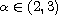in the Riemann-Liouville sense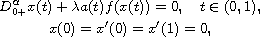and in the Caputo sense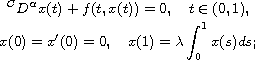and for the third-order differential equation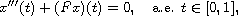associated with three among the following six conditions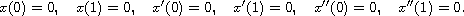Thus, fourteen boundary-value problems at resonance and six boundary-value problems at non-resonanse are studied. Applications of the results are, also, given.

Submitted October 3, 2012. Published June 28, 2013.
Math Subject Classifications: 34B15, 34A10, 34B27, 34B99.
Key Words: Third order differential equation; two-point boundary-value problem; fractional boundary condition; nonexistence of solutions.

Show me the PDF file (334 KB), TEX file, and other files for this article.George L. Karakostas Department of Mathematics University of Ioannina 451 10 Ioannina, Greece email: gkarako@uoi.gr